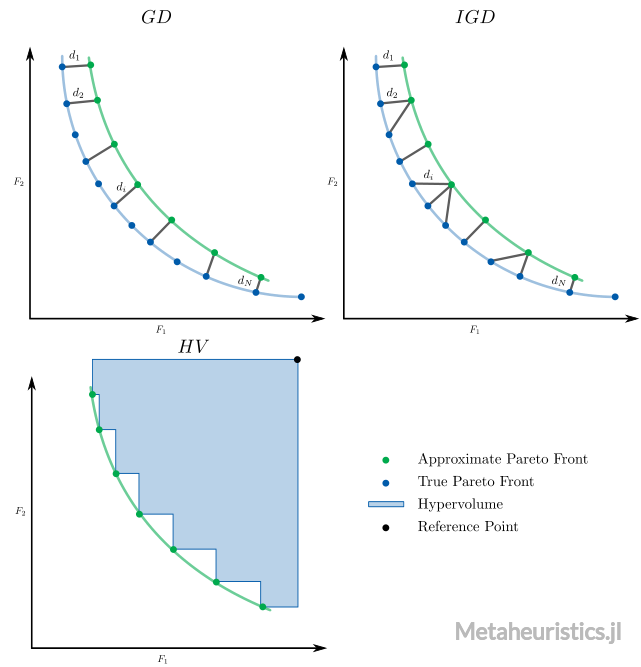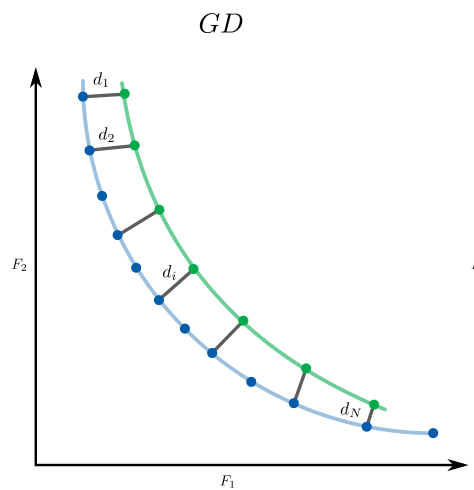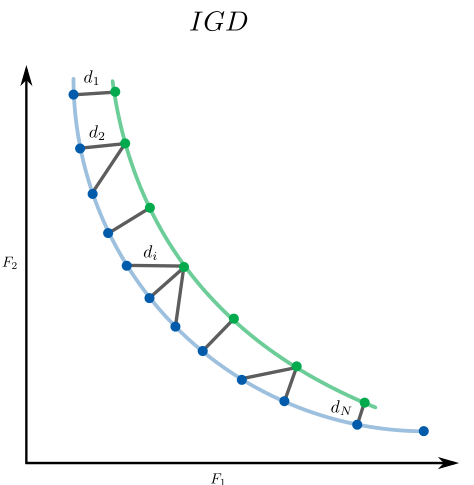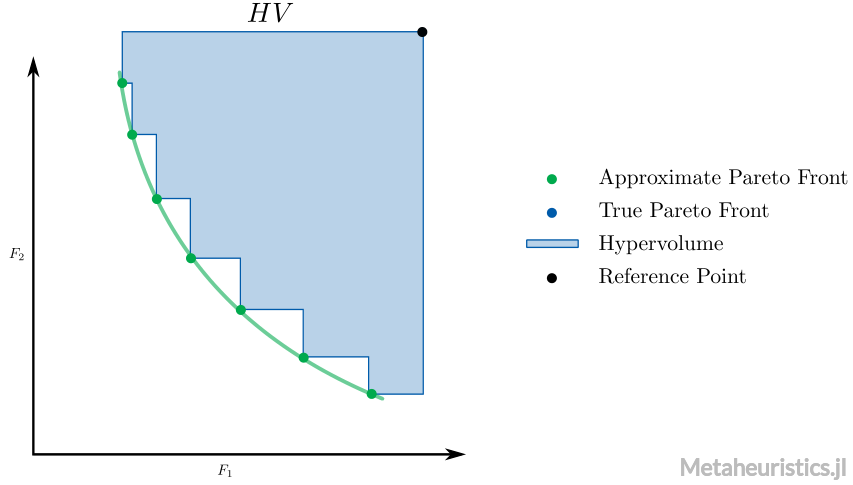# Performance Indicators

Metaheuristics.jl includes performance indicators to assess evolutionary optimization algorithms performance.

Available indicators:Minimization is always assumed here.

Note that in Metaheuristics.jl, minimization is always assumed. Therefore these indicators have been developed for minimization problems.

Metaheuristics.PerformanceIndicatorsModule
PerformanceIndicators

This module includes performance indicators to assess evolutionary multi-objective optimization algorithms.

• gd Generational Distance.
• igd Inverted Generational Distance.
• gd_plus Generational Distance plus.
• igd_plus Inverted Generational Distance plus.
• covering Covering indicator (C-metric).
• hypervolume Hypervolume indicator.

Example

julia> import Metaheuristics: PerformanceIndicators, TestProblems

julia> A = [ collect(1:3) collect(1:3) ]
3×2 Array{Int64,2}:
1  1
2  2
3  3

julia> B = A .- 1
3×2 Array{Int64,2}:
0  0
1  1
2  2

julia> PerformanceIndicators.gd(A, B)
0.47140452079103173

julia> f, bounds, front = TestProblems.get_problem(:ZDT1);

julia> front
F space
┌────────────────────────────────────────┐
1 │⠁⠀⠀⠀⠀⠀⠀⠀⠀⠀⠀⠀⠀⠀⠀⠀⠀⠀⠀⠀⠀⠀⠀⠀⠀⠀⠀⠀⠀⠀⠀⠀⠀⠀⠀⠀⠀⠀⠀⠀│
│⠄⠀⠀⠀⠀⠀⠀⠀⠀⠀⠀⠀⠀⠀⠀⠀⠀⠀⠀⠀⠀⠀⠀⠀⠀⠀⠀⠀⠀⠀⠀⠀⠀⠀⠀⠀⠀⠀⠀⠀│
│⠈⠄⠀⠀⠀⠀⠀⠀⠀⠀⠀⠀⠀⠀⠀⠀⠀⠀⠀⠀⠀⠀⠀⠀⠀⠀⠀⠀⠀⠀⠀⠀⠀⠀⠀⠀⠀⠀⠀⠀│
│⠀⠈⢆⠀⠀⠀⠀⠀⠀⠀⠀⠀⠀⠀⠀⠀⠀⠀⠀⠀⠀⠀⠀⠀⠀⠀⠀⠀⠀⠀⠀⠀⠀⠀⠀⠀⠀⠀⠀⠀│
│⠀⠀⠀⠢⡀⠀⠀⠀⠀⠀⠀⠀⠀⠀⠀⠀⠀⠀⠀⠀⠀⠀⠀⠀⠀⠀⠀⠀⠀⠀⠀⠀⠀⠀⠀⠀⠀⠀⠀⠀│
│⠀⠀⠀⠀⠈⠢⡀⠀⠀⠀⠀⠀⠀⠀⠀⠀⠀⠀⠀⠀⠀⠀⠀⠀⠀⠀⠀⠀⠀⠀⠀⠀⠀⠀⠀⠀⠀⠀⠀⠀│
│⠀⠀⠀⠀⠀⠀⠉⠢⡄⠀⠀⠀⠀⠀⠀⠀⠀⠀⠀⠀⠀⠀⠀⠀⠀⠀⠀⠀⠀⠀⠀⠀⠀⠀⠀⠀⠀⠀⠀⠀│
f_2   │⠀⠀⠀⠀⠀⠀⠀⠀⠈⠑⢤⡀⠀⠀⠀⠀⠀⠀⠀⠀⠀⠀⠀⠀⠀⠀⠀⠀⠀⠀⠀⠀⠀⠀⠀⠀⠀⠀⠀⠀│
│⠀⠀⠀⠀⠀⠀⠀⠀⠀⠀⠀⠈⠲⢄⡀⠀⠀⠀⠀⠀⠀⠀⠀⠀⠀⠀⠀⠀⠀⠀⠀⠀⠀⠀⠀⠀⠀⠀⠀⠀│
│⠀⠀⠀⠀⠀⠀⠀⠀⠀⠀⠀⠀⠀⠀⠈⠒⢤⡀⠀⠀⠀⠀⠀⠀⠀⠀⠀⠀⠀⠀⠀⠀⠀⠀⠀⠀⠀⠀⠀⠀│
│⠀⠀⠀⠀⠀⠀⠀⠀⠀⠀⠀⠀⠀⠀⠀⠀⠀⠈⠙⠢⢄⡀⠀⠀⠀⠀⠀⠀⠀⠀⠀⠀⠀⠀⠀⠀⠀⠀⠀⠀│
│⠀⠀⠀⠀⠀⠀⠀⠀⠀⠀⠀⠀⠀⠀⠀⠀⠀⠀⠀⠀⠀⠈⠑⠢⢄⡀⠀⠀⠀⠀⠀⠀⠀⠀⠀⠀⠀⠀⠀⠀│
│⠀⠀⠀⠀⠀⠀⠀⠀⠀⠀⠀⠀⠀⠀⠀⠀⠀⠀⠀⠀⠀⠀⠀⠀⠀⠈⠉⠢⠤⣀⠀⠀⠀⠀⠀⠀⠀⠀⠀⠀│
│⠀⠀⠀⠀⠀⠀⠀⠀⠀⠀⠀⠀⠀⠀⠀⠀⠀⠀⠀⠀⠀⠀⠀⠀⠀⠀⠀⠀⠀⠀⠉⠑⠢⢤⣀⠀⠀⠀⠀⠀│
0 │⠀⠀⠀⠀⠀⠀⠀⠀⠀⠀⠀⠀⠀⠀⠀⠀⠀⠀⠀⠀⠀⠀⠀⠀⠀⠀⠀⠀⠀⠀⠀⠀⠀⠀⠀⠉⠒⠢⢄⣀│
└────────────────────────────────────────┘
0                                        1
f_1

julia> PerformanceIndicators.igd_plus(front, front)
0.0
source

## Generational DistanceMetaheuristics.PerformanceIndicators.gdFunction
gd(front, true_pareto_front; p = 1)

Returns the Generational Distance.

Parameters

front and true_pareto_front can be:

• N×m matrix where N is the number of points and m is the number of objectives.
• State
• Array{xFgh_indiv} (usually State.population)
source

## Generational Distance Plus

Metaheuristics.PerformanceIndicators.gd_plusFunction
gd_plus(front, true_pareto_front; p = 1)

Returns the Generational Distance Plus.

Parameters

front and true_pareto_front can be:

• N×m matrix where N is the number of points and m is the number of objectives.
• State
• Array{xFgh_indiv} (usually State.population)
source

## Inverted Generational DistanceMetaheuristics.PerformanceIndicators.igdFunction
igd(front, true_pareto_front; p = 1)

Returns the Inverted Generational Distance.

Parameters

front and true_pareto_front can be:

• N×m matrix where N is the number of points and m is the number of objectives.
• State
• Array{xFgh_indiv} (usually State.population)
source

## Inverted Generational Distance Plus

Metaheuristics.PerformanceIndicators.igd_plusFunction
igd_plus(front, true_pareto_front; p = 1)

Returns the Inverted Generational Distance Plus.

Parameters

front and true_pareto_front can be:

• N×m matrix where N is the number of points and m is the number of objectives.
• State
• Array{xFgh_indiv} (usually State.population)
source

## Covering Indicator ($C$-metric)

Metaheuristics.PerformanceIndicators.coveringFunction
covering(A, B)

Computes the covering indicator (percentage of vectors in B that are dominated by vectors in A) from two sets with non-dominated solutions.

A and B with size (n, m) where n is number of samples and m is the vector dimension.

Note that covering(A, B) == 1 means that all solutions in B are dominated by those in A. Moreover, covering(A, B) != covering(B, A) in general.

If A::State and B::State, then computes covering(A.population, B.population) after ignoring dominated solutions in each set.

source

## HypervolumeMetaheuristics.PerformanceIndicators.hypervolumeFunction
hypervolume(front, reference_point)

Computes the hypervolume indicator, i.e., volume between points in front and reference_point.

Note that each point in front must (weakly) dominates to reference_point. Also, front is a non-dominated set.

If front::State and reference_point::Vector, then computes hypervolume(front.population, reference_point) after ignoring solutions in front that do not dominate reference_point.

source

### Examples

Computing hypervolume indicator from vectors in a Matrix

julia> import Metaheuristics.PerformanceIndicators: hypervolumejulia> f1 = collect(0:10); # objective 1julia> f2 = 10 .- collect(0:10); # objective 2julia> front = [ f1 f2 ]11×2 Array{Int64,2}:
0  10
1   9
2   8
3   7
4   6
5   5
6   4
7   3
8   2
9   1
10   0julia> reference_point = [11, 11]2-element Array{Int64,1}:
11
11julia> hv = hypervolume(front, reference_point)66.0

Now, let's compute the hypervolume implementation in Julia from the result of NSGA3 when solving DTLZ2 test problem.

julia> using Metaheuristicsjulia> import Metaheuristics.PerformanceIndicators: hypervolumejulia> import Metaheuristics: TestProblems, get_non_dominated_solutionsjulia> f, bounds, true_front = TestProblems.DTLZ2();julia> result = optimize(f, bounds, NSGA3());julia> approx_front = get_non_dominated_solutions(result.population)100-element Array{Metaheuristics.xFgh_solution{Array{Float64,1}},1}:
(f = [0.5826982323549833, 0.7314005580032928, 0.36835523009765336], g = [0.0], h = [0.0], x = [2.389e-01, 5.717e-01, …, 5.161e-01])
(f = [0.484082007302127, 0.33926718392046085, 0.8111502065936869], g = [0.0], h = [0.0], x = [5.991e-01, 3.892e-01, …, 5.152e-01])
(f = [0.429343998023314, 0.6662489712336719, 0.6231893653107466], g = [0.0], h = [0.0], x = [4.242e-01, 6.356e-01, …, 4.674e-01])
(f = [0.3542572096813144, 0.9322831854054733, 0.09333403980256323], g = [0.0], h = [0.0], x = [5.940e-02, 7.688e-01, …, 4.927e-01])
(f = [1.0012768415285382, 0.06341488869494405, 0.0028311904366864252], g = [0.0], h = [0.0], x = [1.796e-03, 4.027e-02, …, 5.375e-01])
(f = [5.844138530457289e-17, 0.9544202515412942, 0.3021136895136588], g = [0.0], h = [0.0], x = [1.952e-01, 1.000e+00, …, 4.944e-01])
(f = [3.656008541215839e-17, 0.5970715056392251, 0.8065540684662647], g = [0.0], h = [0.0], x = [5.943e-01, 1.000e+00, …, 4.955e-01])
(f = [0.7816133002621791, 0.004158493586445737, 0.6285397101440477], g = [0.0], h = [0.0], x = [4.312e-01, 3.387e-03, …, 4.845e-01])
(f = [0.00013804384184798658, 0.9178848378551203, 0.44175878288866044], g = [0.0], h = [0.0], x = [2.856e-01, 9.999e-01, …, 5.213e-01])
(f = [1.7391551016910975, 0.0, 0.0], g = [0.0], h = [0.0], x = [0.000e+00, 0.000e+00, …, 4.369e-02])
⋮
(f = [0.9476685478616571, 0.3339328262114393, 0.08974316233559428], g = [0.0], h = [0.0], x = [5.671e-02, 2.157e-01, …, 4.803e-01])
(f = [0.15533586754936976, 0.9313693420314089, 0.3339873286416648], g = [0.0], h = [0.0], x = [2.164e-01, 8.948e-01, …, 4.985e-01])
(f = [0.5416089289447406, 0.6908236947763159, 0.48725055265054346], g = [0.0], h = [0.0], x = [3.226e-01, 5.767e-01, …, 4.923e-01])
(f = [0.4679981864763092, 0.5305582750645328, 0.7214462792602528], g = [0.0], h = [0.0], x = [5.062e-01, 5.398e-01, …, 4.813e-01])
(f = [0.20429422627398622, 0.9884556364259565, 0.008272791921642755], g = [0.0], h = [0.0], x = [5.218e-03, 8.703e-01, …, 4.890e-01])
(f = [0.4993752594250251, 0.005988051385664177, 0.8757842282152144], g = [0.0], h = [0.0], x = [6.701e-01, 7.633e-03, …, 4.704e-01])
(f = [0.6108433649147249, 0.7098914076836047, 0.3682171347738249], g = [0.0], h = [0.0], x = [2.385e-01, 5.477e-01, …, 4.813e-01])
(f = [0.15492438021949514, 0.9736422510101419, 0.21880311278467507], g = [0.0], h = [0.0], x = [1.390e-01, 8.995e-01, …, 4.938e-01])
(f = [0.4771542512137947, 0.3253039065636177, 0.8218308441853747], g = [0.0], h = [0.0], x = [6.101e-01, 3.809e-01, …, 5.153e-01])julia> reference_point = nadir(result.population)3-element Array{Float64,1}:
1.7391551016910975
1.0435047298767883
1.0021277671803095julia> hv = hypervolume(approx_front, reference_point)1.2125592879790739

## $\Delta_p$ (Delta $p$)

Metaheuristics.PerformanceIndicators.deltapFunction
deltap(front, true_pareto_front; p = 1)
Δₚ(front, true_pareto_front; p = 1)

Returns the averaged Hausdorff distance indicator aka Δₚ (Delta p).

"Δₚ" can be typed as \Delta<tab>\_p<tab>.

Parameters

front and true_pareto_front can be:

• N×m matrix where N is the number of points and m is the number of objectives.
• Array{xFgh_indiv} (usually State.population)
source

## $\varepsilon$-Indicator

Unary and binary $\varepsilon$-indicator (epsilon-indicator). Details in E. Zitzler, L. Thiele, M. Laumanns, C.M. Fonseca, V.G. da Fonseca (2003)

Metaheuristics.PerformanceIndicators.epsilon_indicatorFunction
epsilon_indicator(A, B)

Computes the ε-indicator for non-dominated sets A and B. It is assumed that all values in A and B are positive. If negative, the sets are translated to positive values.

Interpretation

• epsilon_indicator(A, PF) is unary if PF is the Pareto-optimal front.
• epsilon_indicator(A, B) == 1 none is better than the other.
• epsilon_indicator(A, B) < 1 means that A is better than B.
• epsilon_indicator(A, B) > 1 means that B is better than A.
• Values closer to 1 are preferable.

Examples

julia> A1 = [4 7;5 6;7 5; 8 4.0; 9 2];

julia> A2 = [4 7;5 6;7 5; 8 4.0];

julia> A3 = [6 8; 7 7;8 6; 9 5;10 4.0 ];

julia> PerformanceIndicators.epsilon_indicator(A1, A2)
1.0

julia> PerformanceIndicators.epsilon_indicator(A1, A3)
0.9

julia> f, bounds, pf = Metaheuristics.TestProblems.ZDT3();

julia> res = optimize(f, bounds, NSGA2());

julia> PerformanceIndicators.epsilon_indicator(res, pf)
1.00497701620997
source